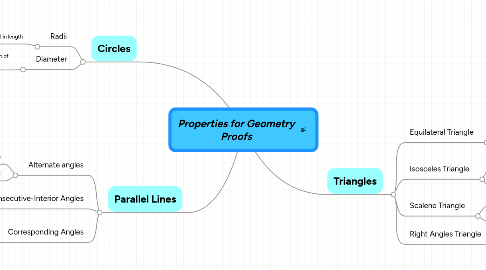# Properties for Geometry Proofs

Lists the geometrical properties needed for congruency proofs.

Get Started. It's FreeProperties for Geometry Proofs## 1. Circles

1.1.1. Are always equal in length

### 1.2. Diameter

1.2.1. Always double the length of the radius

## 2. Triangles

### 2.1. Equilateral Triangle

2.1.1. Three equal sides

2.1.2. Three equal angles

### 2.2. Isosceles Triangle

2.2.1. Two sides equal

2.2.2. Angles opposite equal sides equal (base angles)

### 2.3. Scalene Triangle

2.3.1. No equal sides

2.3.2. No equal angles

### 2.4. Right Angles Triangle

2.4.1. One angle is a right angle

## 3. Parallel Lines

### 3.1. Alternate angles

3.1.1. Form a Z shape

3.1.2. Are always equal

### 3.2. Consecutive-Interior Angles

3.2.1. Form a C shape

3.2.2. Are Supplmentary

### 3.3. Corresponding Angles

3.3.1. Form a F shape

3.3.2. Are always equal